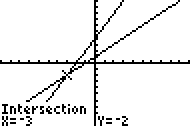# Knowledge Base

## Solution 11958: Finding the Intersection of Two Functions on the TI-83 Family, TI-84 Plus Family, or TI-Nspire™ Handheld in TI-84 Plus Mode Graphing Calculators.

### How can I find the intersection of two functions on the TI-83 family, TI-84 Plus family or TI-Nspire Handheld in TI-84 Plus Mode graphing calculators?

The intersect operation (selectable from the CALCULATE menu) finds the coordinates of a point at which two or more functions intersect using solve().  The intersection must appear on the display to use intersect.

Refer to the following example in order to calculate the intersection of the graphs of two functions.

Example:

Find the intersection of the following functions:
y = x + 1
y = 2x + 4

Solution:

1. Graph the functions:
• Press [Y=] to display the Y= Editor.
• Input x+1 next to Y1=.
• Input 2x+4 next to Y2=.
• Press [GRAPH] to display the graphs of the two functions.

2. Find the intersection of the two lines:
• Press [2nd] [CALC]  to select 5:interesect from the CALCULATE menu.
• When First curve? is displayed, press the up and down directional keys, if necessary, to move the cursor to the first function, then press [ENTER].

• When Second curve? is displayed, press the up and down directional keys, if necessary, to move the cursor to the second function, then press [ENTER].
NOTE: The calculator requires that a first and second curve be selected in case there are more than two functions being graphed at one time.  This is to specify which two functions to find the intersection of, in that case.

• When Guess? is displayed, use the left and right directional keys to move the cursor to the point that is your guess as to the location of the intersection, and then press [ENTER].
NOTE: The calculator requires that a guess be made in case the functions being graphed intersect more than once.  This is to specify which intersection of the two selected functions to find, in that case.

The cursor is on the solution and the coordinates are displayed, even if CoordOff format is selected.  Intersection is displayed in the bottom-left corner.  To restore the free-moving cursor, press any of the directional keys.For additional instructions on performing graph calculations, please see the TI-83 family and TI-84 Plus family guidebooks for additional information.

Note: TI-Nspire users may refer to the TI-84 Plus family guidebook